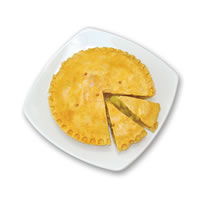# MathsClass

A blog about teaching and learning in a maths classroom.

## Sector Graphs

Thursday, 12 February 2009 | 3 Comments

In my fourth year of teaching, I’m finally happy with how teaching sector graphs went.Teaching Yr 8 to draw sector (pie) graphs sounds easy, yet has proven difficult. In producing a sector graph, there are a couple fundamentals drawn together — and connecting seemingly disparate concepts presents a roadblock for many students at our school.

Here’s the sequence of lessons that I used this year:

1. drawing circles and dividing those circles up into sectors given angles. For example, draw the sector graph for the following: Red 180°, Blue 90°, Green 45° and Yellow 45°. Now this may seem simple, but this lesson covers:
• using a compass
• using a protractor
• using a 180° protractor to draw an angle greater than 180°
• labelling and giving a title to a sector graph
• checking your work by making sure that the angles drawn complete the circle (add to 360°)
• find fractions of a circle, i.e. determining a sector angle giving a fraction. Then applying this to drawing sector graphs. For example, given data like the colour of MP3 Players sold: White 12, Silver 13 and Black 16, draw a sector graph. In this lesson:
• 360° in a circle gets repeated and repeated
• we find fractions of 360° by dividing by the denominator (and multiplying by the numerator if we have a fraction like 512)
1. in lesson 3, we finally draw a sector graph from scratch given raw data. Mind you, the raw data is “nice” – that is, number of people surveyed might be 360, 36, 18, 24, 300 or 1800, which all give nice sector angles. In this lesson, I introduce a scaffold (a table) for manipulating the data prior to drawing a sector graph. For example:

Eye Colour Number Fraction Angle Size
Blue 10 1024 150°
Brown 8 824 120°
Hazel 6 624 90°
Total 24

This is where I saw students start to just take to drawing the sector graph with minimal guidance, suggesting they’d “got it”.

1. in the fourth lesson, we applied these new found skills to critique movie posters. Credit here to Dan Meyer for sharing his lesson Pie Charts – Movie Posters. I changed the worksheets a little, including making an instruction sheet that included tables for completing the working out. This was a good lesson, because we had to deal with some problems as a class. Some students didn’t rank every poster, meaning students had different totals to work with (some got 17, some 18… and some the correct 21). These don’t all translate into nice sector angles meaning we had to think about dealing with fractions of a degree (ok, so we basically ignored them and fudged the angles).

Four lessons which seemed to graduate nicely, and as we move on to the next type of graph, sector graphs seem complete.

By the way, Rocky seemed to be the most popular poster.

Image credit: Pie Chart by net_efekt on Flickr (Creative Commons)

Posted in • Lesson IdeaGraphs | Short URL: http://mths.co/1397

• Share:

Tim on  13 February 09  at  03:08 AM #
Why are they called sector graphs instead of pie charts? Does this confuse your students? For the particular example you have given in lesson one, a protractor is not necessary. It can be done with a compass and a straight edge.

author

Simon on  13 February 09  at  11:09 PM #
Hi Tim, Just checked the Syllabus, which calls them "sector (pie) graphs" and later on just "sector graphs". I use both terms in class, and at one point suggest they should be called "pizza graphs". I don't think there's confusion, I probably get 2 questions when we go to a computer lab and use Excel which calls them "pie graphs". In Lesson one, that example is a bit easy, unless your straight edge also has a right angle, you might use a set square. But anyway, we do a few other examples too. Often things we do in class can be done a simpler way, but I always teach them a way that always works, if they find a simpler way, great. So, even though that example is easy, it's still worth practising with a protractor.
Tim on  14 February 09  at  07:30 AM #
Interesting that the syllabus calls them sector graphs, I have never heard them called that in the "real world". Drawing 45 and 90 degree angles with a compass and a straight edge isn't necessarily easier than using a protractor. I just like using geometry.

Commenting is not available in this channel entry.

New  Subscribe to the …

Simon Job — eleventh year of teaching maths in a public high school in Western Sydney, Australia.
MathsClass is about teaching and learning in a maths classroom. more→

## Elsewhere@simonjob
updates via@mathslinks